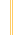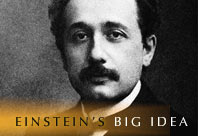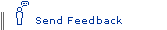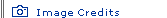# The Power of Tiny ThingsEinstein's Big Idea homepage

Have you ever thought about the power of a paper clip? If you could convert the mass of a paper clip entirely to energy, how big a punch would it pack? Einstein's most famous equation, E = mc2, indicates the calculation is straightforward: the energy released would be equivalent to the mass times the speed of light squared. Yet the answer is far from intuitive because, for one thing, the speed of light is immense—670 million mph—making the speed of light squared almost inconceivable. In this quiz, discover the answer and explore other examples of what scientists call mass-energy equivalence.—Susan K. Lewis

1. The mass of a small paper clip (.03 ounces) has the energy of:

1. burning 20 gallons of gasoline
2. one ton of TNT
3. an atomic bomb

2. In 1939, physicist Lise Meitner realized that splitting a single atom of uranium would effectively convert the mass of one-fifth of a proton into 200 million electron volts of energy. This is enough energy to:

1. kill an amoeba
2. boil 43 gallons of room-temperature water
3. launch the Space Shuttle

3. A vast amount of solar energy warms our planet and supports life. If all that energy were converted to mass, how much mass would strike Earth in a single second?

1. 12 ounces
2. four pounds
3. 32 pounds

4. The mass of 100 pennies has just enough energy to:

1. melt a pound of lead
2. power New York State for about two days
3. power our entire world for a month

5. If converted entirely to energy, a lump of coal weighing 2.2 pounds could power a 100-watt light bulb for how long?

1. 67 hours
2. 29 years
3. 29 million years

6. The mass of a house has the energy to:

1. drive a large SUV around the world
2. melt half the ice in Antarctica
3. possibly split Earth in half

7. Just as mass can become energy, energy can become mass. When the Space Shuttle travels at its top speed, the energy of motion adds to its mass. The amount of gain is roughly comparable to:

1. a flea
2. a stick of butter
3. a cantaloupe